# 简单说，正则表达式是…

python中必备的工具，主要是用来查找和匹配字符串的。

# 正则表达式怎么用❓

``import re``

## sreach的用法????

### 匹配连续的多个数值????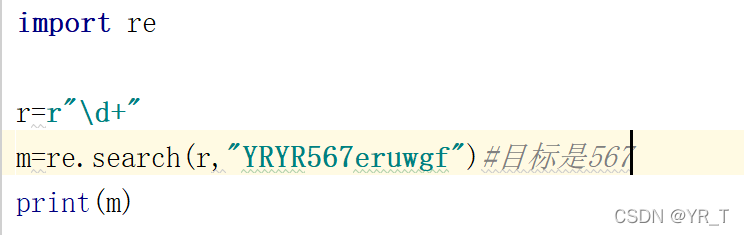import re
r=r”\d+”
m=re.search(r,”YRYR567eruwgf”)#目标是567
print(m)

re模块中，r“\d+”正则表达式表示匹配连续的多个数值，search是re中的函数,从”YRYR567eruwgf”字符串中搜索连续的数值,得到”567″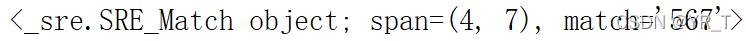### 字符”+”重复前面一个匹配字符一次或者多次????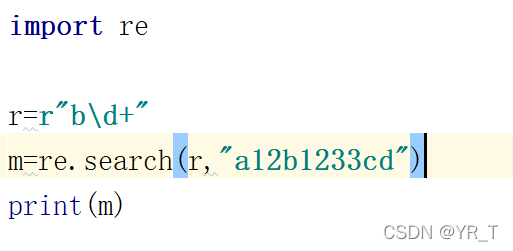import re
r=r”b\d+”
m=re.search(r,”a12b1233cd”)
print(m)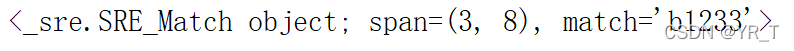### 字符”*”重复前面一个匹配字符零次或者多次????

“*” 与 “+”类似,但有区别,列如:

“匹配的是”a”,因为表示”b”可以重复零次，但是”+“却要求”b”重复一次以上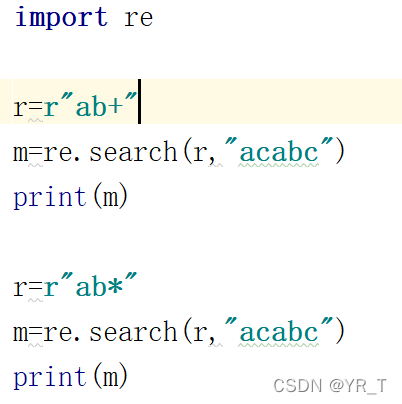import re
r=r”ab+”
m=re.search(r,”acabc”)
print(m)
r=r”ab*”
m=re.search(r,”acabc”)
print(m)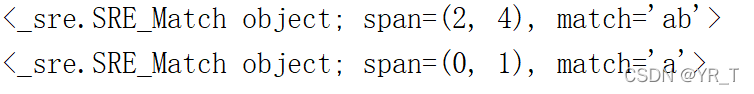### 字符”?”重复前面一个匹配字符零次或者一次????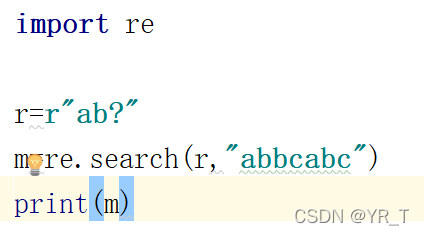import re
r=r”ab?”
m=re.search(r,”abbcabc”)
print(m)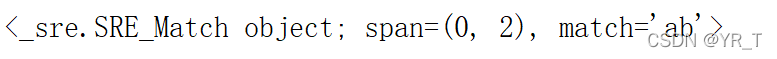###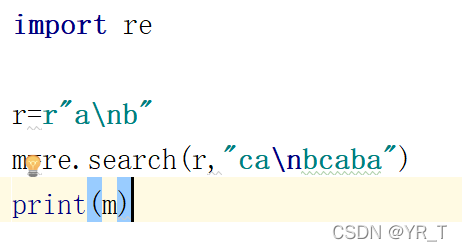import re
r=r”a\nb”
m=re.search(r,”ca\nbcaba”)
print(m)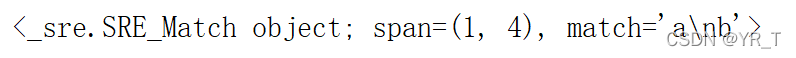### 完整表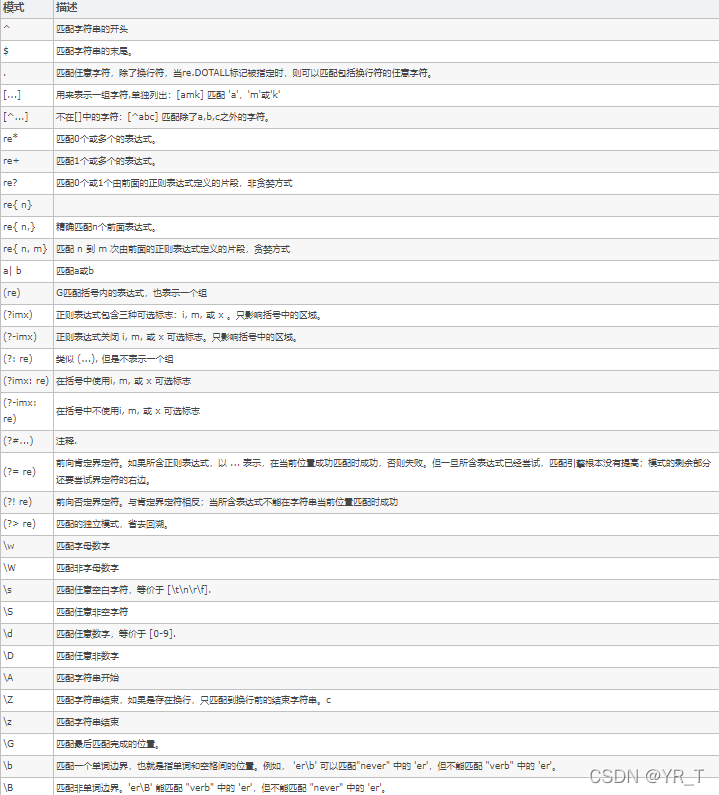## match用法

### match用法

#示例：
name=’张三’
if re.match(‘张\w+’,name):
print(‘{},你好！’.format(name))
# 张三,你好！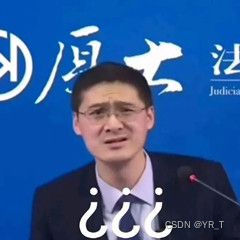• 返回匹配的match对象
• 默认从给定字符串的开头开始匹配、即使正则表达式没有用^声明匹配开头

### match对象

Match对象的几个属性:

1..string 待匹配的文本
2..re 匹配使用的pattern对象
3..pos 正则表达式搜索文本的开始位置
4..endpos 正则表达式搜索文本的结束位置
Match对象的几个方法:
1.group(0) 返回匹配到的子串
2.start() 返回匹配子串的开始位置
3.end() 返回匹配子串的结束位置
4.span() 返回start()、end()

### 数量词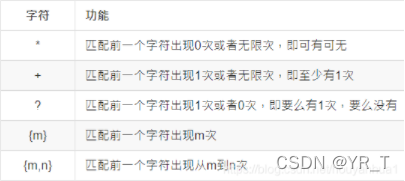### 匹配分组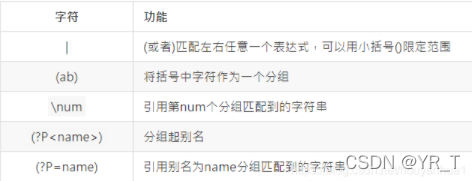### match总结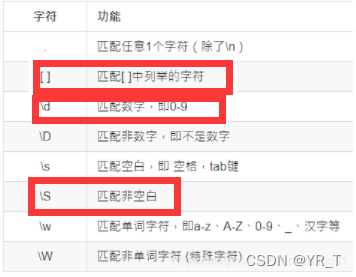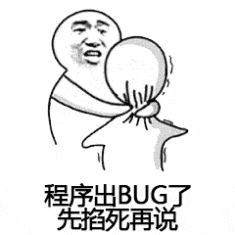# 实践出真知 凸(｀0´)凸 ☀️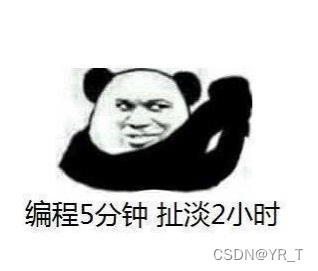ε=(´ο｀*)))唉，还是自己写吧、、、

import phone
from time import *
import re
def begin():
print(“欢迎来到查询小程序“)
print(“1.查询”)
print(“2.用户”)
def p(n):
if re.match(r’1[3,4,5,7,8]\d{9}’, n):
if re.match(r’13[0,1,2]\d{8}’, n) or \
re.match(r”15[5,6]\d{8}”, n) or \
re.match(r”18[5,6]”, n) or \
re.match(r”145\d{8}”, n) or \
re.match(r”176\d{8}”, n):
return True
elif re.match(r”13[4,5,6,7,8,9]\d{8}”, n) or \
re.match(r”147\d{8}|178\d{8}”, n) or \
re.match(r”15[0,1,2,7,8,9]\d{8}”, n) or \
re.match(r”18[2,3,4,7,8]\d{8}”, n):
return True
else:
return True
else:
return False
if __name__ == “__main__”:
s=0
begin()
while True:
op = int(input(“请输入:”))
if op==1:
phoneNum = str(input(“请输入你的电话号码”))
if p(phoneNum)==False:
print(“该手机号无效”)
for i in range(100):
print(‘\n’)
begin()
else:
info = phone.Phone().find(phoneNum)
print(“手机号码:”+str(info[“phone”]))
print(“手机所属地:”+str(info[“province”])+”省”+str(info[“city”])+”市”)
print(“邮政编号:”+str(info[“zip_code”]))
print(“区域号码:”+str(info[“area_code”]))
print(“手机类型:”+str(info[“phone_type”]))
s+=1
i = input(“输入任意数退出…”)
for i in range(100):
print(‘\n’)
begin()
if op==2:
print(“使用次数:”+str(s))
i = input(“输入任意数退出…”)
for i in range(100):
print(‘\n’)
begin()# 写在最后